# Sharpe Ratio

## A Sharper Sharpe III : MLEs

In a previous blog post we looked at Damien Challet's 'Sharper estimator' of the Signal-Noise Ratio, confirming that it exhibits substantially lower standard error (and usually root mean square error) than the traditional 'moment-based' estimator known as the Sharpe ratio. Those simulations used returns drawn from a shifted, scaled $$t$$ distribution, which is symmetric. In a followup post, we looked at returns drawn from a Lambert W x Gaussian distribution, which has substantial skew. The very design of the drawdown estimator suggests it will be biased for skewed returns, an artifact which was visible in that study.

What if we viewed the drawdown estimator instead as a proof of concept? That is, you heard (on the streets, if you will) that there is a more efficient estimator of the Signal Noise ratio for shifted, scaled $$t$$ variates; your job is to recreate that estimator. How would you proceed? Here I perform that thought experiment, though in a limited setting. (Part of the intrigue of the drawdown estimator is that it is not an obvious result of this gedankenexperiment, so far is it from 'usual' statistical practice, for better or for worse.)

For this study we will pretend that $$x_i = \mu + \sqrt{1 - (2/\nu)} t_i$$, where $$t_i$$ are independent draws from a $$t$$ distribution with $$\nu \ge 4$$ degrees of freedom. Note that the variance of $$x_i$$ is exactly 1, so the mean is equal to the Signal to Noise ratio. We will look at estimators of the mean of $$x$$, but compare them against the drawdown estimator, which is at a strict disadvantage here.

## MLE

The first approach that comes to mind is Maximum Likelihood Estimation. MLEs are asymptotically efficient, though they are not terribly robust to model misspecification.

The likelihood of a single observation is

$$\frac{1}{\left(\frac{1}{\nu - 2} \left(\nu + \left(\mu - x_i\right)^{2} - 2\right)\right)^{\frac{\nu}{2} + \frac{1}{2}} \sqrt{- \nu + 2} \beta{\left (\frac{1}{2},\frac{\nu}{2} \right )}}$$

The log likelihood of $$n$$ observations is

$$\mathcal{L} = c\left(\nu\right) - \frac{\nu+1}{2} \sum_i \log{\left(1 + \frac{\left(\mu - x_i\right)^2}{\nu - 2}\right)}.$$

Again we are assuming $$\nu$$ is known a priori, which is an odd simplifying assumption. The first order conditions for the maximum likelihood estimator of $$\mu$$ (i.e. take the derivative and set to zero) yield the recusive definition of $$\mu$$:

$$\mu = \frac{\sum_i w_i x_i}{\sum_i w_i},\,\mbox{where}\, w_i = \frac{1}{1 + \frac{\left(\mu - x_i\right)^2}{\nu - 2}}.$$

In other words, a weighted mean where observations farther from $$\mu$$ are downweighted.

We could estimate $$\mu$$ iteratively via this equation, but it is simpler to just pass the log likelihood and its derivative functions to a numerical solver made for this purpose.

We will also need the Fisher Information, which is based on the second derivative of the log likelihood with respect to $$\mu$$. This takes the value:

$$\mathcal{L}_{\mu,\mu} = \sum_i \frac{\left(\nu + 1\right) \left(- \nu + \left(\mu - x_i\right)^{2} + 2\right)}{\left(\nu + \left(\mu - x_i\right)^{2} - 2\right)^{2}}.$$

To compute the Fisher Information we need the expected value of that thing. Sympy is happy to compute that expectation, but yields an awful expression of no use. Instead we will estimate the expectation numerically. We then take one over negative that expectation to get the Cramér Rao Lower Bound on the variance of an unbiased estimator of $$\mu$$. In our case it will be some multiple of $$1/n$$.

Here is some code to compute these things:

library(maxLik)

# log likelihood of
# ((x - mu) / sqrt(1-(2/nu))) ~ t(df=nu)
simp_tlik <- function(param,x,nu) {
mu <- param
sg <- sqrt(1 - (2/nu))
t <- (x-mu) / sg
sum(dt(t,df=nu,log=TRUE) - log(sg))
}
# gradient of same
simp_tlikgrad <- function(param,x,nu) {
mu <- param
sg <- sqrt(1 - (2/nu))
t <- (x-mu) / sg
n <- length(x)
gradmu <- ((nu+1)/2) * sum(2 * t / (1 + (t^2)/nu))
gradmu
}

simp_mle_est <- function(x,nu) {
param0 <- c(mean(x))
foo <- maxLik(logLik=simp_tlik,grad=simp_tlikgrad,x=x,nu=nu,start=param0)
param <- foo$estimate param } # compute n times the cramer rao lower bound, numerically crlb <- function(nu,nsim=500000) { tv <- rt(n=nsim,df=nu) a11 <- (nu * (nu+1) / (nu-2)) * mean( (tv^2 - nu) / ((nu+tv^2)^2) ) fishi <- - a11 crbnd <- 1 / fishi }  Here is a plot of $$n$$ times the Cramér Rao lower bound as a function of $$\nu$$: suppressMessages({ library(dplyr) library(tidyr) library(ggplot2) }) params <- data.frame(nu=3:15) %>% group_by(nu) %>% summarize(crlbval=crlb(nu)) %>% ungroup() ph <- params %>% ggplot(aes(nu,crlbval)) + geom_line() + geom_point() + scale_x_sqrt() + labs(x=expression(paste(nu,' the degrees of freedom of the t distribution')), y='n times the Cramer Rao lower bound') print(ph)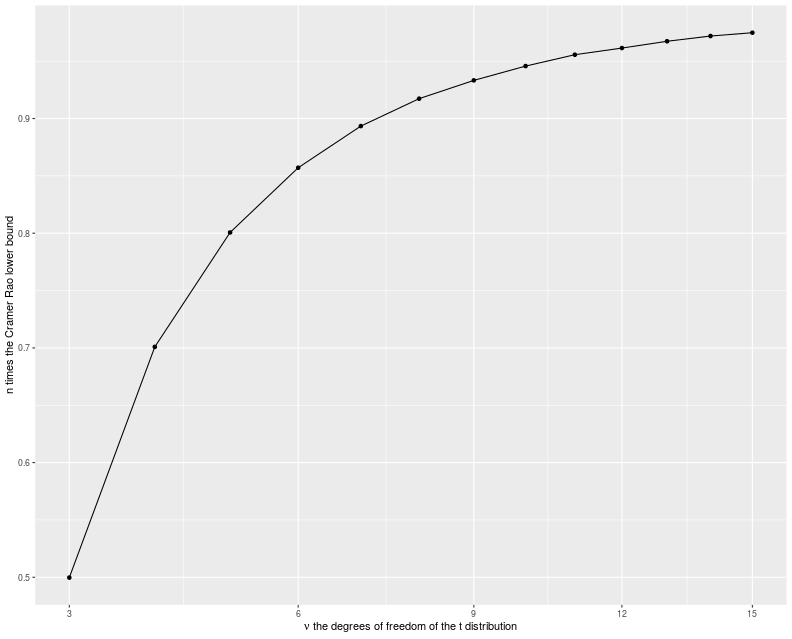## Trimmed mean The other estimator we will try is the trimmed mean. First assume some $$\gamma$$ between 0 and $$1/2$$ is chosen. Then compute the $$\gamma$$ and $$1-\gamma$$ sample quantiles of the observed $$x_i$$. Take the mean of the subset of the $$x_i$$ which are between those two quantiles (there are approximately $$n\left(1 - 2\gamma\right)$$ such $$x_i$$). As $$\gamma \to 1/2$$ you should recover the sample median, while as $$\gamma \to 0$$ you get the (untrimmed) sample mean. It turns out that the sample trimmed mean has a standard error of approximately $$\frac{s_w}{\left(1-2\gamma\right)\sqrt{n}},$$ where $$s_w$$ is the square root of the Winsorized variance. (Approximately here means there is an extra term that is of the order $$1/n$$.) Winsorizing is like trimming, but you cap the values rather than throw them away. That is define $$\hat{x} = \max{\left(q_{\gamma},\min{\left(q_{1-\gamma},x\right)}\right)}$$, where $$q_{\alpha}$$ is the population $$\alpha$$ quantile of the distribution of $$x$$. Then the variance of $$\hat{x}$$ is the Winsorized variance of $$x$$. For our toy problem, since we know the density of $$x$$ we can easily estimate the Winsorized variance, and thus we can find the $$\gamma$$ that gives the smallest standard error. (c.f. John Cook's post on the efficiency of the median versus the mean for $$t$$ variates.) Here is some code to compute these things: suppressMessages({ library(dplyr) library(tidyr) library(magrittr) }) trim_x <- function(x,alpha=0.2,beta=1-alpha) { stopifnot(alpha > 0, alpha < 0.5,beta > 0.5, beta < 1) # trimmed mean qs <- quantile(x,c(alpha,beta)) tx <- x[(x >= min(qs)) & (x <= max(qs))] } trim_mean <- function(x,alpha=0.2,beta=1-alpha) { mean(trim_x(x,alpha=alpha,beta=beta)) } # find the standard error of the gamma-trimmed mean on a t variable # find the approximate standard error of the gamma-trimmed mean on a # shifted, scaled$t$distribution. tse <- function(gamma,nu,ncut=9999) { stopifnot(gamma > 0,gamma < 0.5) sg <- sqrt(1 - (2/nu)) tlims <- qt(c(gamma,1-gamma),df=nu) xs <- sg * seq(from=min(tlims),to=max(tlims),length.out=ncut) delx <- diff(xs) ys <- xs^2 * (1/sg) * dt(xs/sg,df=nu) # compute the Winsorized variance: winsv <- 0.5 * (sum(ys[-1] * delx) + sum(ys[-ncut] * delx)) + gamma * sum((sg*tlims)^2) sqrt(winsv) / (1 - 2 * gamma) } # find the optimal trimming for a t_nu random variable min_tes <- function(nu,eps=0.0001,ncut=9999) { f <- function(x) tse(x,nu=nu,ncut=ncut) ys <- optimize(f, interval=c(eps,0.5-eps)) ys$minimum
}


Here is a plot of the approximately optimal $$\gamma$$ as a function of $$\nu$$:

suppressMessages({
library(dplyr)
library(tidyr)
library(ggplot2)
})

params <- data.frame(nu=unique(c(seq(4.01,4.09,by=0.01),
seq(4.1,5.0,by=0.1),
seq(5.25,10,by=0.25),
seq(11,20,by=1),
seq(22,50,by=2),
seq(55,100,by=5)))) %>%
group_by(nu) %>%
summarize(gamma=min_tes(nu)) %>%
ungroup()

ph <- params %>%
ggplot(aes(nu,gamma)) +
geom_point() + stat_smooth(method='lm') + scale_x_sqrt() + scale_y_log10() +
labs(x=expression(paste(nu,', the degrees of freedom of the t distribution')),
y=expression(paste('the ',gamma,' which gives the lowest standard error for the trimmed mean')))
print(ph)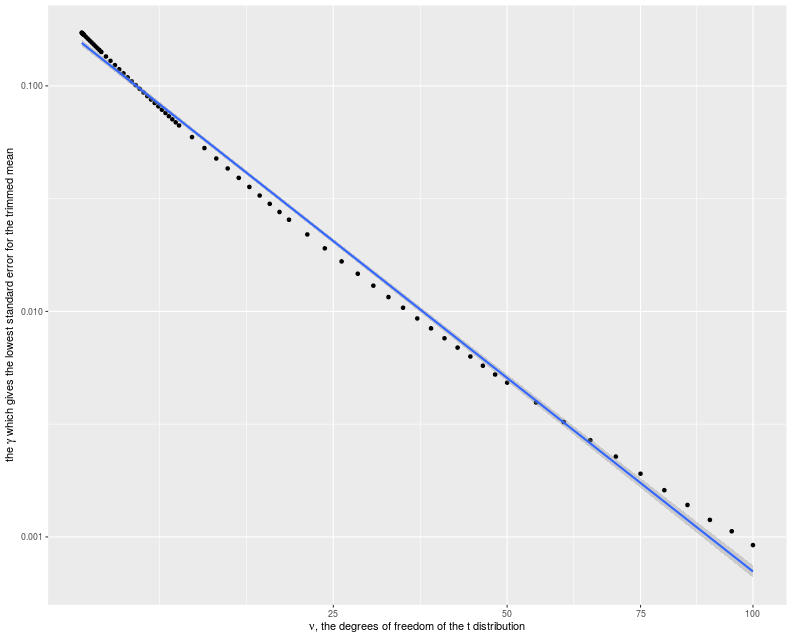We should like to know how efficient the trimmed mean is compared to the Cramér Rao bound. So here we assume a sample size of, say 252, and compute both approximate standard error of the optimally trimmed mean, and the Cramér Rao bound. We see that they are very close to each other.

n <- 252
params <- data.frame(nu=unique(c(seq(4.01,4.09,by=0.01),
seq(4.1,5.0,by=0.1),
seq(5.25,10,by=0.25),
seq(11,20,by=1),
seq(22,50,by=2),
seq(55,100,by=5)))) %>%
group_by(nu) %>%
summarize(gamma=min_tes(nu)) %>%
ungroup() %>%
group_by(nu,gamma) %>%   # add on the estimated tse
mutate(trim_se=tse(gamma,nu)) %>%
ungroup() %>%   # add on the crlb
group_by(nu) %>%
mutate(crbit=sqrt(crlb(nu=nu))) %>%
ungroup() %>%
mutate(trim_se = trim_se / sqrt(n),
crlb = crbit / sqrt(n)) %>%
dplyr::select(-crbit)

ph <- params %>%
tidyr::gather(key=series,value=value,trim_se,crlb) %>%
mutate(series=case_when(.$series=='trim_se' ~ 'approximate standard error of trimmed mean', .$series=='crlb'    ~ 'Cramer Rao lower bound',
TRUE ~ 'bad code')) %>%
ggplot(aes(nu,value,color=series)) +
geom_line() +
scale_x_log10() +
labs(x=expression(paste(nu,', the degrees of freedom of the t distribution')),
y='estimator of standard error',
title='comparison of trimmed mean standard error to the lower bound')
print(ph)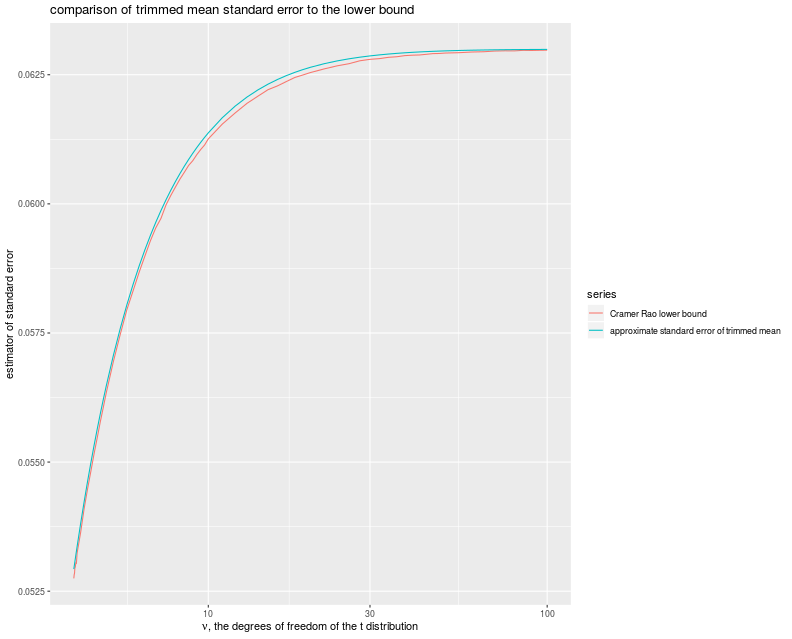## Simulations

Let's throw all these things together into some simulations. We will draw variates from a $$t$$ distribution with $$\nu$$ degrees of freedom known, rescale to unit variance, then add on $$\mu$$. We will then compute the MLE of the mean, and the trimmed mean with near optimal trimming. To make things interesting, we will also compute the drawdown estimator of Signal Noise ratio, even though it is at a disadvantage, since it must estimate the volatility and the degrees of freedom. We will compute the mean and the Sharpe ratio for reference. We will also compute, as a function of $$\nu$$, the Cramér Rao bound and the approximate theoretical standard error of the trimmed mean, for reference:

suppressMessages({
library(dplyr)
library(tidyr)
library(tibble)
library(sharpeRratio)
library(maxLik)
library(moments)
# https://cran.r-project.org/web/packages/doFuture/vignettes/doFuture.html
library(doFuture)
registerDoFuture()
plan(multiprocess)
})

# only works for scalar pzeta:
onesim <- function(n,pzeta,nu,gamma,...) {
x <- pzeta + sqrt(1 - (2/nu)) * rt(n,df=nu)
mux <- mean(x)
sdx <- sd(x)
mome <- mux / sdx
trym <- trim_mean(x,alpha=gamma)
mlee <- simp_mle_est(x,nu=nu)
ddsr <- unlist(lapply(pzeta-pzeta,function(addon) {
sim <- sharpeRratio::estimateSNR(x+addon)
sim$SNR })) cbind(pzeta,mux,mome,ddsr,mlee,trym) } repsim <- function(nrep,n,pzeta,nu,gamma) { dummy <- invisible(capture.output(jumble <- replicate(nrep,onesim(n=n,pzeta=pzeta,nu=nu,gamma=gamma)),file='/dev/null')) retv <- aperm(jumble,c(1,3,2)) dim(retv) <- c(nrep * length(pzeta),dim(jumble)) colnames(retv) <- colnames(jumble) invisible(as.data.frame(retv)) } manysim <- function(nrep,n,pzeta,nu,gamma,nnodes=5) { if (nrep > 2*nnodes) { # do in parallel. nper <- table(1 + ((0:(nrep-1) %% nnodes))) retv <- foreach(i=1:nnodes,.export = c('n','pzeta','nu','gamma','onesim','repsim')) %dopar% { repsim(nrep=nper[i],n=n,pzeta=pzeta,nu=nu,gamma=gamma) } %>% bind_rows() } else { retv <- repsim(nrep=nrep,n=n,pzeta=pzeta,nu=nu,gamma=gamma) } retv } ope <- 252 pzetasq <- c(0,1/4,1,4) / ope pzeta <- sqrt(pzetasq) nus <- tibble::tribble(~nu,4.2,4.75,7,16) # get bounds bounds <- nus %>% group_by(nu) %>% # figure out optimal gamma mutate(gamma=min_tes(nu,eps=0.0001)) %>% ungroup() %>% group_by(nu,gamma) %>% # add on the estimated tse mutate(trim_se=tse(gamma,nu)) %>% ungroup() %>% # add on the crlb group_by(nu) %>% mutate(crbit=sqrt(crlb(nu=nu))) %>% ungroup() params <- tidyr::crossing(tibble::tribble(~n,128,256,512,1024), tibble::tibble(pzeta=pzeta), nus) %>% left_join(bounds,by='nu') %>% mutate(trim_se = trim_se / sqrt(n)) %>% mutate(crbound = crbit / sqrt(n)) %>% dplyr::select(-crbit) # run a bunch nrep <- 500 set.seed(1234) system.time({ results <- params %>% group_by(n,pzeta,nu,gamma,trim_se,crbound) %>% summarize(sims=list(manysim(nrep=nrep,nnodes=8, pzeta=pzeta,n=n,nu=nu,gamma=gamma))) %>% ungroup() %>% tidyr::unnest() %>% dplyr::select(-pzeta1) })   user system elapsed 5560.71 2864.67 1247.19  Now I collect the results of the simulation, computing the bias, standard error and root mean square error of the two methods of computing Sharpe, and joining on with the population moment values. # summarize the results sumres <- results %>% tidyr::gather(key=stat,value=value,-n,-nu,-gamma,-pzeta,-trim_se,-crbound) %>% mutate(error=value - pzeta) %>% group_by(n,nu,gamma,pzeta,stat,trim_se,crbound) %>% summarize(mean=mean(value,na.rm=TRUE), bias=mean(error,na.rm=TRUE), sd=sd(value,na.rm=TRUE), rmse=sqrt(mean(error^2,na.rm=TRUE))) %>% ungroup() %>% tidyr::gather(key=metric,value=value,mean,bias,sd,rmse) %>% tidyr::spread(key=stat,value=value) %>% mutate(ref=mome) %>% tidyr::gather(key=stat,value=value,mux,mome,ddsr,mlee,trym) %>% mutate(ratio=value / ref) %>% mutate(stat=case_when(.$stat=='mome' ~ 'moment based Sharpe',
.$stat=='ddsr' ~ 'drawdown based SNR estimator', .$stat=='mux'  ~ 'vanilla mean',
.$stat=='mlee' ~ 'MLE of mean', .$stat=='trym' ~ 'optimal trim mean',
TRUE ~ 'bad code')) %>%
mutate(annualized SNR=signif(pzeta * sqrt(ope),digits=2)) %>%
mutate(ex_kurt=6 / (nu - 4)) %>%
rename(excess kurtosis=ex_kurt) %>%
mutate(excess kurtosis=signif(excess kurtosis,digits=3))


Now I plot the ratio of the empirical RMSEs to the Cramér Rao lower bound versus the number of days of data, with facet columns for $$\nu$$ (equivalently, the excess kurtosis), and facet rows for the Signal to Noise ratio:

ph <- sumres %>%
filter(metric=='rmse') %>%
dplyr::select(-ratio,-trim_se) %>%
mutate(ratio=value / crbound) %>%
mutate(annualized SNR=factor(annualized SNR)) %>%
ggplot(aes(n,ratio,color=stat)) +
geom_line() + geom_jitter(width=0,height=0.01,alpha=0.5) +
scale_x_log10() + scale_y_sqrt() +
geom_hline(yintercept=1,linetype=2,alpha=0.5) +
facet_grid(annualized SNR~excess kurtosis+nu,labeller=label_both) +
labs(y='ratio of empirical RMSE to the Cramer Rao lower bound',
x='number of days of data',
title='ratio of empirical RMSE to Cramer Rao lower bound, t returns')
print(ph)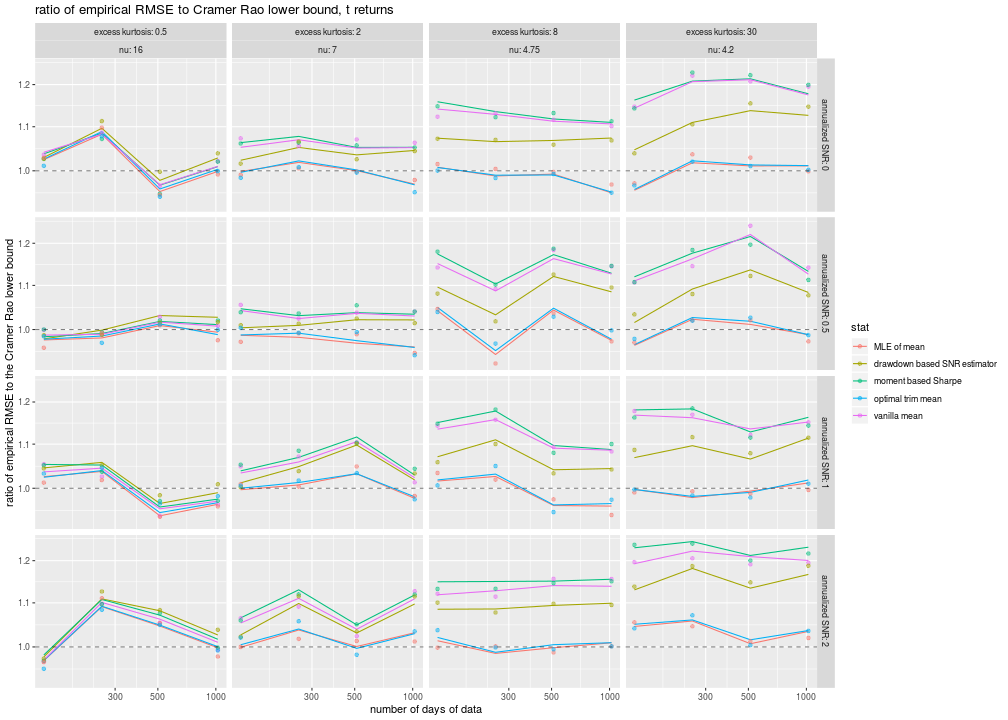A few conclusions from this plot, in increasing order of surprise:

• The trimmed mean and the MLE of the mean have nearly indistinguishable RMSE. I have jittered the points slightly in the hope of making the points visible.
• The trimmed mean outperforms the vanilla mean in terms of lower RMSE, with the more noticeable effect for more leptokurtic distributions on the right. The trimmed mean nearly achieves the lower bound (and is sometimes a little less, probably due to sampling variation).
• The vanilla mean and the moment-based Sharpe have nearly the same RMSE. That is, the estimation of the volatility contributes very little to the total error.
• The drawdown estimator, despite having two hands tied behind its back, achieves lower RMSE than the Cramér Rao lower bound. This 'super efficiency' is most visible for low Signal-Noise, high kurtosis situations, as in the upper right corner of our plots. For higher SNR, the bias of the drawdown estimator takes over, and the drawdown estimator underperforms even the sample mean.

What? How does an estimator achieve a lower RMSE than the Cramér Rao lower bound? Especially one that is operating at a loss, estimating more than is necessary for the CRLB? Recall that one of the technical conditions of the Cramér Rao theory is that the distribution of errors of the estimator must be independent of the parameter being estimated. I call this the 'no stopped clocks' condition: just as a stopped clock is right twice a day, an estimator which is biased towards certain values can seem to be superefficient when tested near those values. I mentioned in a previous post that I suspect the drawdown estimator is employing a kind of 'unconscious shrinkage', as it has been trained on data which have Signal Noise ratio around -1 to 1 or so in annualized terms. The simulations here strongly suggest this kind of shrinkage. Practitioners should exercise extreme caution before using the drawdown estimator!

## Honest Sharpe simulations

Above we performed simulations to estimate the mean of returns with known volatility and kurtosis. An 'honest' estimator does not know these in advance. As above, I create a trimmed mean Sharpe, which estimates the $$\nu$$ parameter by computing the excess kurtosis, then uses the optimal $$\gamma$$ for that $$\nu$$. As we expect little noise from the volatility estimate, we simply normalize by the vanilla sample standard deviation. We also implement an MLE estimator which assumes a shifted, scaled $$t$$ distribution, but estimates the $$\nu, \sigma$$ and $$\mu$$ separately. We test these, and the vanilla Sharpe ratio and the drawdown estimator, on $$t$$ returns, but also on skewed returns from a Lambert W x Gaussian distribution. Here are the simulations:

suppressMessages({
library(dplyr)
library(tidyr)
library(tibble)
library(sharpeRratio)
library(maxLik)
library(moments)
# https://cran.r-project.org/web/packages/doFuture/vignettes/doFuture.html
library(doFuture)
registerDoFuture()
plan(multiprocess)
})

# log likelihood of
# (x - mu) / sg ~ t(df=nu)
tlik <- function(param,x) {
mu <- param
sg <- param
nu <- max(4,param)
t <- (x-mu) / sg
## also equal to:
#n <- length(x)
#retv <- n * (lgamma((nu+1)/2) - lgamma(nu/2)- 0.5 * log(nu * pi) - log(sg)) - ((nu+1)/2) * sum(log(1 + (t^2)/nu))
sum(dt(t,df=nu,log=TRUE) - log(sg))
}
# gradient of same
tlikgrad <- function(param,x) {
mu <- param
sg <- param
nu <- max(4,param)
t <- (x-mu) / sg

n <- length(x)
gradmu <- ((nu+1)/2) * sum(2 * t / (1 + (t^2)/nu))
gradsg <- - (n / sg) + ((nu+1)/2) * sum(2 * t / ((sg^2) * (1 + (t^2)/nu)))
gradnu <- (n/2) * (digamma((nu+1)/2) - digamma(nu/2) - (1/nu)) - (1/2) * sum(log(1 + (t^2)/nu)) + ((nu+1)/2) * sum(((t^2)/(n^2)) / (1 + (t^2)/nu))
c(gradmu,gradsg,gradnu)
}

mle_est <- function(x) {
exk <- moments::kurtosis(x) - 3
nuest <- 4 + 6/max(0.0001,exk)
param0 <- c(mean(x),sd(x),nuest)
A <- matrix(c(0,1,0,0,0,1),nrow=2,byrow=TRUE)
B <- matrix(c(0,-4),nrow=2)
cons <- list(ineqA = A,ineqB = B)
foo <- maxLik(logLik=tlik,grad=tlikgrad,x=x,start=param0,constraints=cons,method='BFGS')
# now compute 'sharpe'
param <- foo$estimate truesg <- param * sqrt(param / (param-2)) param / truesg } trim_x <- function(x,alpha=0.2,beta=1-alpha) { stopifnot(alpha > 0, alpha < 0.5,beta > 0.5, beta < 1) # trimmed mean qs <- quantile(x,c(alpha,beta)) tx <- x[(x >= min(qs)) & (x <= max(qs))] } trim_mean <- function(x,alpha=0.2,beta=1-alpha) { mean(trim_x(x,alpha=alpha,beta=beta)) } trim_sharpe <- function(x,alpha=0.2,beta=1-alpha) { # trimmed mean tmu <- trim_mean(x,alpha=alpha,beta=beta) # 2FIX: also compute standard errors? retv <- tmu / sd(x) } # find the standard error of the gamma-trimmed mean on a t variable # find the approximate standard error of the gamma-trimmed mean on a # shifted, scaled$t$distribution. tse <- function(gamma,nu,ncut=9999) { stopifnot(gamma > 0,gamma < 0.5) sg <- sqrt(1 - (2/nu)) tlims <- qt(c(gamma,1-gamma),df=nu) xs <- sg * seq(from=min(tlims),to=max(tlims),length.out=ncut) delx <- diff(xs) ys <- xs^2 * (1/sg) * dt(xs/sg,df=nu) # compute the Winsorized variance: winsv <- 0.5 * (sum(ys[-1] * delx) + sum(ys[-ncut] * delx)) + gamma * sum((sg*tlims)^2) sqrt(winsv) / (1 - 2 * gamma) } # find the optimal trimming for a t_nu random variable min_tes <- function(nu,eps=0.0001,ncut=9999) { f <- function(x) tse(x,nu=nu,ncut=ncut) ys <- optimize(f, interval=c(eps,0.5-eps)) ys$minimum
}
opt_gammas <- data.frame(nu=unique(c(seq(4.01,4.09,by=0.005),
seq(4.1,5.0,by=0.05),
seq(5.25,10,by=0.25),
seq(11,20,by=1),
seq(22,50,by=2),
seq(55,100,by=5)))) %>%
group_by(nu) %>%
summarize(gamma=min_tes(nu)) %>%
ungroup() %>%
mutate(ex_kurt=6 / (nu - 4))

opt_trim_sharpe <- function(x) {
exk <- moments::kurtosis(x) - 3
if (exk <= min(opt_gammas$ex_kurt)) { # vanilla sharpe retv <- mean(x) / sd(x) } else { if (exk <= max(opt_gammas$ex_kurt)) {
best <- approx(x=opt_gammas$ex_kurt,y=opt_gammas$gamma,xout=exk)
gam <- best$y } else { gam <- max(opt_gammas$gamma)
}
retv <- trim_sharpe(x,alpha=gam)
}
retv
}

onesim <- function(n,pzeta,gen=rnorm,...) {
# works on one pzeta
x <- gen(n) + pzeta
mome <- mean(x) / sd(x)
trym <- opt_trim_sharpe(x)
mlee <- mle_est(x)
ddsr <- sharpeRratio::estimateSNR(x)$SNR cbind(pzeta,mome,ddsr,mlee,trym) } repsim <- function(nrep,n,pzeta,gen=rnorm) { dummy <- invisible(capture.output(jumble <- replicate(nrep,onesim(n=n,pzeta=pzeta,gen=gen)),file='/dev/null')) retv <- aperm(jumble,c(1,3,2)) dim(retv) <- c(nrep * length(pzeta),dim(jumble)) colnames(retv) <- colnames(jumble) invisible(as.data.frame(retv)) } manysim <- function(nrep,n,pzeta,gen=rnorm,nnodes=5) { if (nrep > 2*nnodes) { # do in parallel. nper <- table(1 + ((0:(nrep-1) %% nnodes))) retv <- foreach(i=1:nnodes,.export = c('n','pzeta','gen','onesim','repsim')) %dopar% { repsim(nrep=nper[i],n=n,pzeta=pzeta,gen=gen) } %>% bind_rows() } else { retv <- repsim(nrep=nrep,n=n,pzeta=pzeta,gen=gen) } retv } tgen <- function(df) { rescal <- sqrt((df - 2)/df) function(n) { rescal * rt(n,df=df) } } #Lambert x Gaussian gen_lambert_w <- function(n,dl = 0.1,mu = 0,sg = 1) { require(LambertW,quietly=TRUE) force(dl) force(mu) force(sg) Gauss_input = create_LambertW_input("normal", beta=c(0,1)) params = list(delta = c(0), gamma=c(dl), alpha = 1) LW.Gauss = create_LambertW_output(Gauss_input, theta = params) #get the moments of this distribution moms <- mLambertW(beta=c(0,1),distname=c("normal"),delta = 0,gamma = dl, alpha = 1) if (!is.null(LW.Gauss$r)) {
# API changed in 0.5:
samp <- LW.Gauss$r(n=n) } else { samp <- LW.Gauss$rY(params)(n=n)
}
samp <- mu  + (sg/moms$sd) * (samp - moms$mean)
}
lamgen <- function(delta) {
lapply(delta,function(del) { function(n) gen_lambert_w(n=n,dl=del,mu=0,sg=1) })
}
moms_lambert_w <- function(dl = 0.1,mu = 0,sg = 1) {
require(LambertW,quietly=TRUE)
Gauss_input = create_LambertW_input("normal", beta=c(0,1))
params = list(delta = c(0), gamma=c(dl), alpha = 1)
LW.Gauss = create_LambertW_output(Gauss_input, theta = params)
#get the moments of this distribution
moms <- mLambertW(beta=c(0,1),distname=c("normal"),delta = 0,gamma = dl, alpha = 1)
moms$mean <- mu moms$sd <- sg
return(as.data.frame(moms))
}

generators <- tibble::tribble(~name, ~gen, ~skewness, ~ex_kurt,
'gaussian',  rnorm,   0,  0,
't_6',     tgen(6),   0, 6 / (6-4),
't_4.75',  tgen(4.75),   0, 6 / (4.75-4),
't_4.1',  tgen(4.1),   0, 6 / (4.1-4)) %>%
rbind( tibble::tribble(~delta,0.012,0.25,0.5) %>%
group_by(delta) %>%
summarize(moms=list(moms_lambert_w(dl=delta))) %>%
ungroup() %>%
mutate(name=paste0('lambert_',delta)) %>%
unnest(moms) %>%
mutate(gen=(lamgen(delta))) %>%
mutate(ex_kurt=kurtosis-3) %>%
dplyr::select(-mean,-sd,-kurtosis,-delta)
)

ope <- 252
pzetasq <- c(0,1/4,1,4) / ope
pzeta <- sqrt(pzetasq)

params <- tidyr::crossing(tibble::tribble(~n,128,256,512,1024),
tibble::tibble(pzeta=pzeta),
generators)

# run a bunch
nrep <- 250
set.seed(1234)
system.time({
results <- params %>%
group_by(n,pzeta,name,skewness,ex_kurt) %>%
summarize(sims=list(manysim(nrep=nrep,nnodes=8,
pzeta=pzeta,n=n,gen=gen[]))) %>%
ungroup() %>%
tidyr::unnest() %>%
dplyr::select(-pzeta1)
})

   user  system elapsed
9732.12 2510.39 1867.67


Now I collect the results of the simulation, computing the bias, standard error and root mean square error of the two methods of computing Sharpe, and joining on with the population moment values.

# summarize the results.
sumres <- results %>%
tidyr::gather(key=stat,value=value,-n,-name,-skewness,-ex_kurt,-pzeta) %>%
mutate(error=value - pzeta) %>%
group_by(n,name,skewness,ex_kurt,pzeta,stat) %>%
summarize(mean=mean(value,na.rm=TRUE),
bias=mean(error,na.rm=TRUE),
sd=sd(value,na.rm=TRUE),
rmse=sqrt(mean(error^2,na.rm=TRUE))) %>%
ungroup() %>%
tidyr::gather(key=metric,value=value,mean,bias,sd,rmse) %>%
tidyr::spread(key=stat,value=value) %>%
mutate(ref=mome) %>%
tidyr::gather(key=stat,value=value,mome,ddsr,mlee,trym) %>%
mutate(ratio=value / ref) %>%
mutate(stat=case_when(.$stat=='mome' ~ 'moment based estimator', .$stat=='ddsr' ~ 'drawdown based estimator',
.$stat=='mlee' ~ 'MLE based estimator', .$stat=='trym' ~ 'optimal trim mean estimator',
TRUE ~ 'bad code')) %>%
mutate(annualized SNR=signif(pzeta * sqrt(ope),digits=2)) %>%
mutate(skewness=signif(skewness,digits=3)) %>%
mutate(asymmetric=!grepl('^(gauss|t_\\d)',name)) %>%
rename(excess kurtosis=ex_kurt) %>%
mutate(excess kurtosis=signif(excess kurtosis,digits=3))


Now I plot the ratio of the empirical RMSE to that of the moment-based estimator, and plot versus the number of days of data, with facet rows for the annualized Signal Noise ratio and columns for the skew and excess kurtosis of the distribution. I then also plot the bias of all four estimators

# plot rmses
ph <- sumres %>%
filter(metric=='rmse') %>%
filter(!grepl('moment based',stat)) %>%
mutate(annualized SNR=factor(annualized SNR)) %>%
ggplot(aes(n,ratio,color=stat)) +
geom_line() + geom_point() +
scale_x_log10() + scale_y_log10() +
geom_hline(yintercept=1,linetype=2,alpha=0.5) +
facet_grid(annualized SNR~asymmetric+skewness+excess kurtosis,labeller=label_both) +
labs(y='ratio of empirical RMSE to that of moment estimator',
x='number of days of data',
title='ratio of empirical RMSE ratio estimators of Signal Noise ratio, various returns distributions')
print(ph)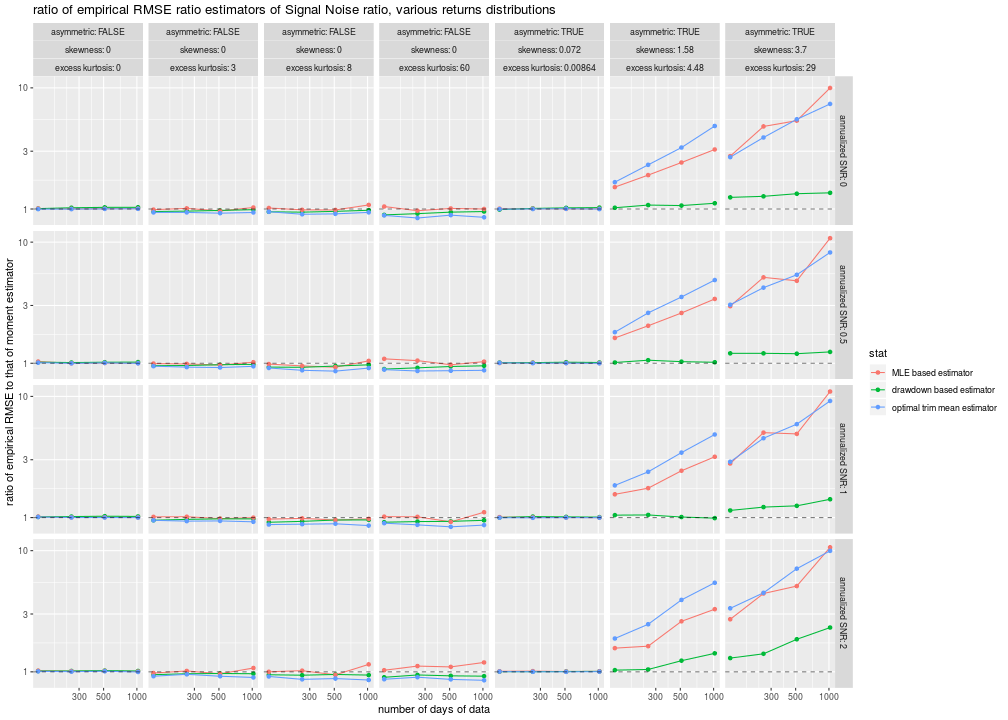# plot bias
ph <- sumres %>%
filter(metric=='bias') %>%
mutate(annualized SNR=factor(annualized SNR)) %>%
ggplot(aes(n,value,color=stat)) +
geom_line() + geom_point() +
scale_x_log10() +
geom_hline(yintercept=0,linetype=2,alpha=0.5) +
facet_grid(annualized SNR~skewness+excess kurtosis,labeller=label_both) +
labs(y='empirical bias',
x='number of days of data',
title='empirical bias of estimators of Signal Noise ratio, various returns distribugtions')
print(ph)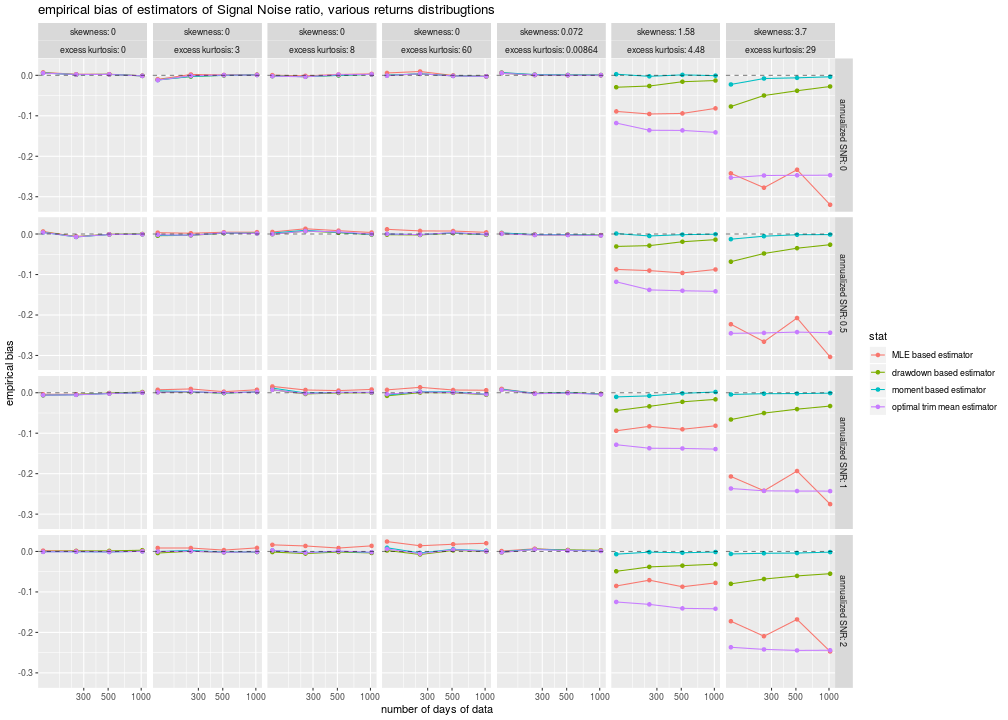Some observations:

• For the symmetric distributions, the trimmed Sharpe outperforms the MLE, which only does about as well as the vanilla Sharpe. Neither of them gives the performance of the dradown estimator, except for the high SNR case, perhaps due to shrinkage.
• Both the trimmed Sharpe and the MLE estimators are awful for skewed distributions, much worse than the drawdown estimator, and are horribly biased. They simply should not be used.

The question remains: can we improve on the vanilla Sharpe to deal with higher order moments, while avoiding bias, keeping some theoretical guarantees, and not shrinking to zero?

atom feed · Copyright © 2018-2019, Steven E. Pav.
The above references an opinion and is for information purposes only. It is not intended to be investment advice. Seek a duly licensed professional for investment advice.